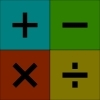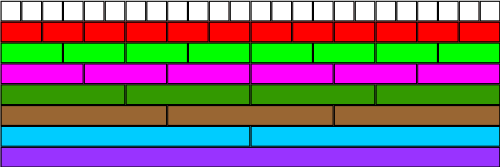#### You may also like### Worms

Place this "worm" on the 100 square and find the total of the four squares it covers. Keeping its head in the same place, what other totals can you make?### Which Scripts?

There are six numbers written in five different scripts. Can you sort out which is which?### Highest and Lowest

Put operations signs between the numbers 3 4 5 6 to make the highest possible number and lowest possible number.

##### Age 7 to 11Challenge Level

You may like to try our Fractional Wall problem before this one.Using the fraction wall above, can you say which is bigger, $\frac{1}{3}$ or $\frac{2}{8}$? By how much?

Which is smaller, $\frac{5}{6}$ or $\frac{3}{4}$? By how much?

What is the difference between $\frac{5}{6}$ and $\frac{1}{3}$?

What is three quarters of $\frac{2}{3}$? Can you explain how you worked this out?

Having a visual representation of fractions as a wall will aid children's understanding of both equivalent fractions and comparisons of fractions, but perhaps it will also equip them with a method to help them in the future. It is important that pupils have an appreciation of what is being taken as "the whole" and this may need some discussion before the problem as it stands is tackled. Using real Cuisenaire rods to aid manipulation of fractions is invaluable, although the lengths pose some restrictions. However, OHT rods could be used as a way into the problem by concentrating on just a few different lengths to start with.

This could be used to help older children to understand the concepts necessary for manipulating fractions to add and subtract them. It is amazing how much easier the visualisation makes the arithmetic process. Just try it yourself and see.